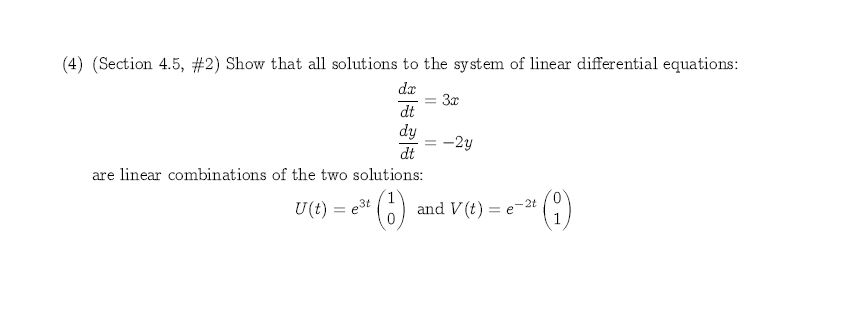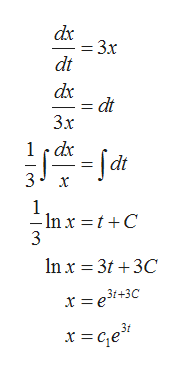(4) (Section 4.5, #2) Show that all solutions to the system of linear differential equations:dr3xdtdy-2ydtare linear combinations of the two solutions:and V (t)- 2tU(t) e3t

Question

It is linear algebra. Please see the attachmenthelp_outlineImage Transcriptionclose(4) (Section 4.5, #2) Show that all solutions to the system of linear differential equations: dr 3x dt dy -2y dt are linear combinations of the two solutions: and V (t) - 2t U(t) e3t fullscreen
Step 1

Given system of first order linear differential equations is shown below

Step 2

To show that all solutions of given system of linear differential equations can be written as a linear combination of below two solution.

Step 3

Solution of given differential eq...help_outlineImage Transcriptionclosedx = 3x dt dx dt 3х 1dx 3 x 1 In x =t +C 3 In x 3t3C x =e3+3C fullscreen

Want to see the full answer?

See Solution

Want to see this answer and more?

Our solutions are written by experts, many with advanced degrees, and available 24/7

See Solution
Tagged in

Other